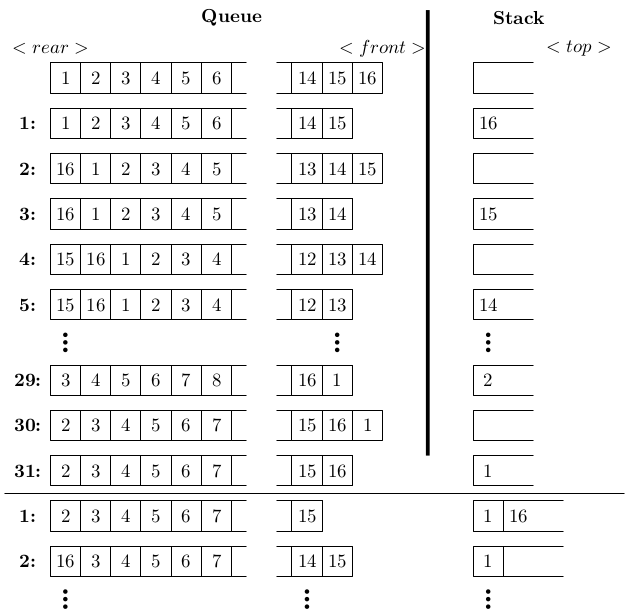##### number of iteration in stack

Let Q denote a queue containing sixteen numbers and S be an empty stack. Head(Q) returns the element at the head of the queue Q without removing it from Q. Similarly Top (S) returns the element at the top of S without removing it from S. Consider the algorithm given below.

while Q is not Empty do
if S is Empty OR Top(S) ≤ Head (Q) then
x:= Dequeue (Q);
Push (S, x);
else
x:= Pop(S);
Enqueue (Q, x);
end
end

The maximum possible number of iterations of the while loop in the algorithm is _______.

Arvind Rawat
9 Jun 2016 08:29 pm

The maximum possible number of iterations will be n2 which occurs when the numbers are stored in increasing order in Q. It can be proved by taking an example. Take the initial Q as 8,4,2,1. Now execute the given code.

Ranita Biswas
11 Jun 2016 02:29 pm

If you follow the execution for a given queue with increasing order of elements from rear to front, you will get something like this:Now, see that for each element (except the last or smallest one), we once push it into the stack and in next iteration again pop it from the stack and enqueue at the rear of queue. This is the worst case of execution. So, we do (2n - 1) = 2*16 - 1 = 31 iterations to finally stabilize the smallest element in the stack. After this 31st iteration, we get back to the same situation as the initial input except the number of elements in the queue has now been decreased to 15. So, we can calculate that the total number of maximum possible iterations are:
(2n - 1) + (2(n-1) - 1) + (2(n-2) - 1) + ... (2(n-(n-1)) - 1)
= 2(n + (n-1) + (n-2) + ... + 1) - n
= 2n(n+1)/2 - n
= n(n+1) - n
= n2
= 162 = 256

Arvind Rawat
11 Jun 2016 02:26 pm

The approach is absolutely correct. However, there is an error in the calculation. Sum of first n terms is n(n+1)/2 :).

Ranita Biswas
11 Jun 2016 02:29 pm

Thanks Arvind. Now corrected.

Arvind Rawat
11 Jun 2016 02:24 pm

Sorry didnt get the time to explain the example suggested. Here it follows:

First push 8  pop 8, push 4 pop 4, push 2 pop 2, push1.
After that push 8 pop 8, push 4 pop 4, push 2.
Again push 8 pop 8, push 4.
Finally push 8.

So, at each step we can see that a pattern is followed and if we relate it with n, then we get 2n-1, 2(n-1)-1, 2(n-2)-1 .... 2(1)-1 respectively. So, adding all these terms, we get
2(n + n-1 + n-2 +......+ 1) - n = 2(n(n+1)/2) - n = n2 + n - n = n2.

abhinandan
11 Jun 2016 03:39 pm

thanks alot arvind sir nd ranita mam for the explanation

Sutanay Bhattacharjee
20 Jul 2018 10:14 am
why while loop runs for maximum no of times when input is in descending order